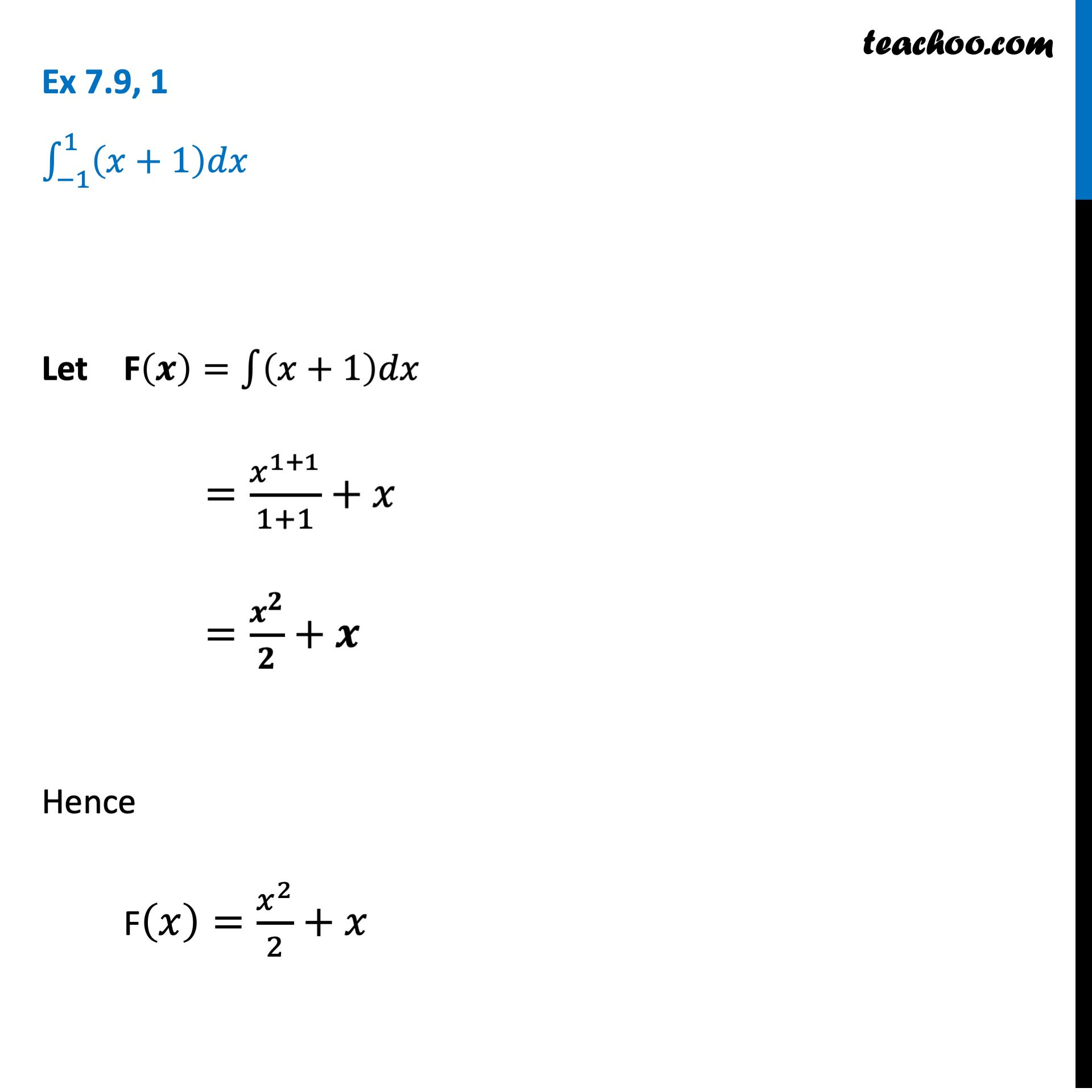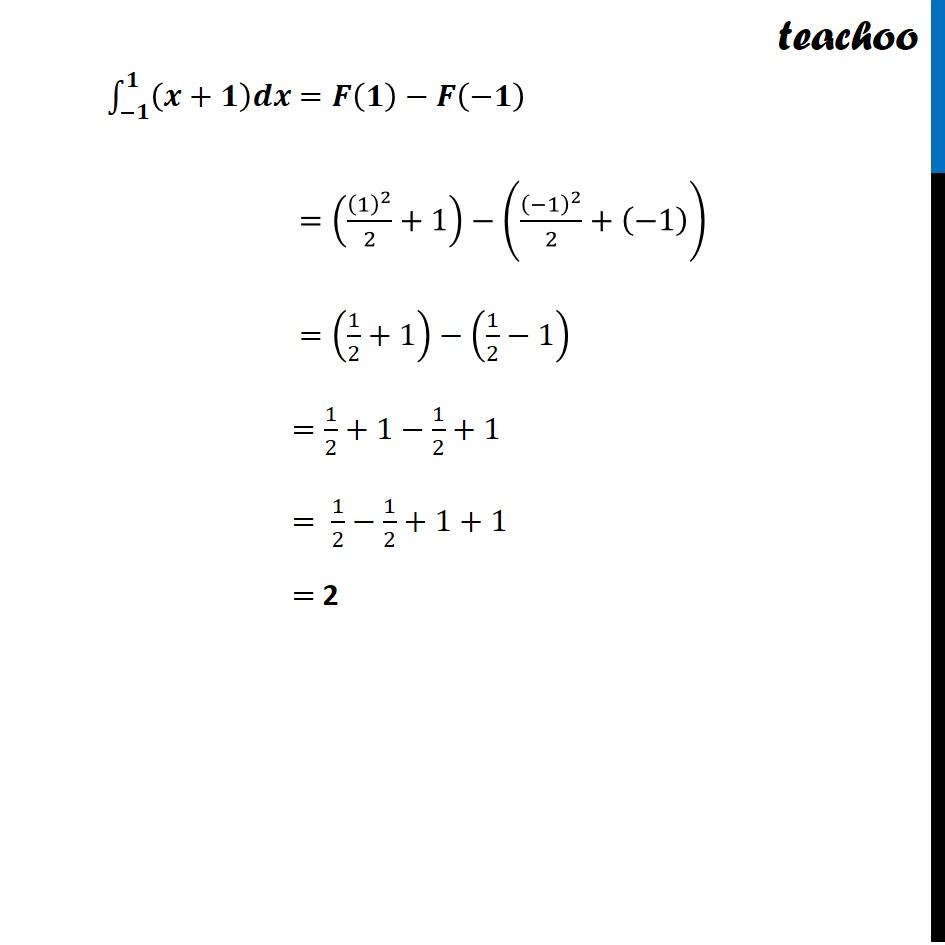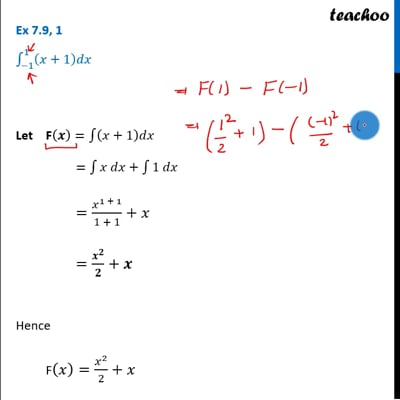Definite Integration - By Formulae

Chapter 7 Class 12 Integrals
Concept wiseThis video is only available for Teachoo black users

Solve all your doubts with Teachoo Black (new monthly pack available now!)

### Transcript

Ex 7.9, 1 ∫_(−1)^4▒(𝑥+1)𝑑𝑥 Let F(𝒙)=∫1▒(𝑥+1)𝑑𝑥 =∫1▒〖𝑥 𝑑𝑥〗 +∫1▒〖1 𝑑𝑥〗 =𝑥^(1+1)/(1+1)+𝑥 =𝒙^𝟐/𝟐+𝒙 Hence F(𝑥)=𝑥^2/2+𝑥 ∫_(−𝟏)^𝟏▒(𝒙+𝟏)𝒅𝒙 =𝑭(𝟏)−𝑭(−𝟏) =((1)^2/2+1)−((−1)^2/2+(−1)) =(1/2+1)−(1/2−1) =1/2+1−1/2+1 = 1/2−1/2+1+1 = 2